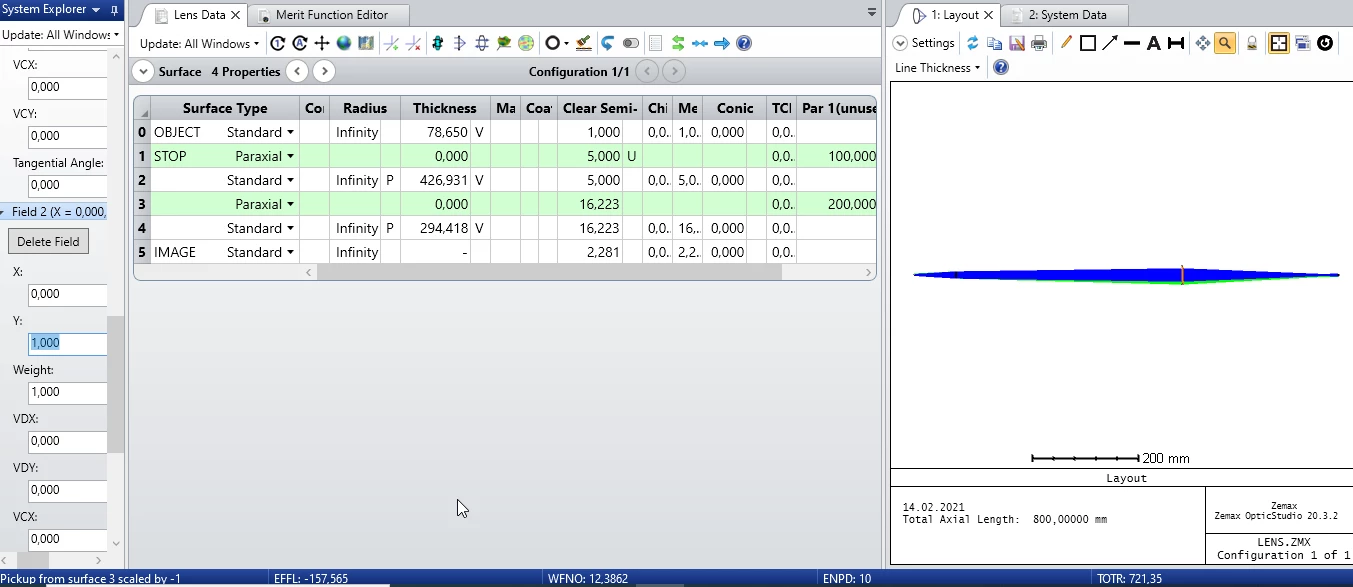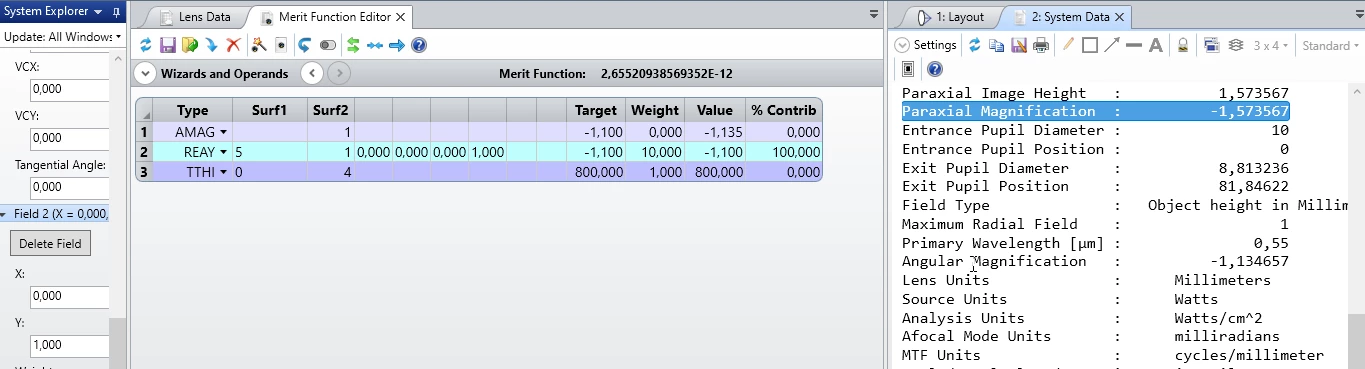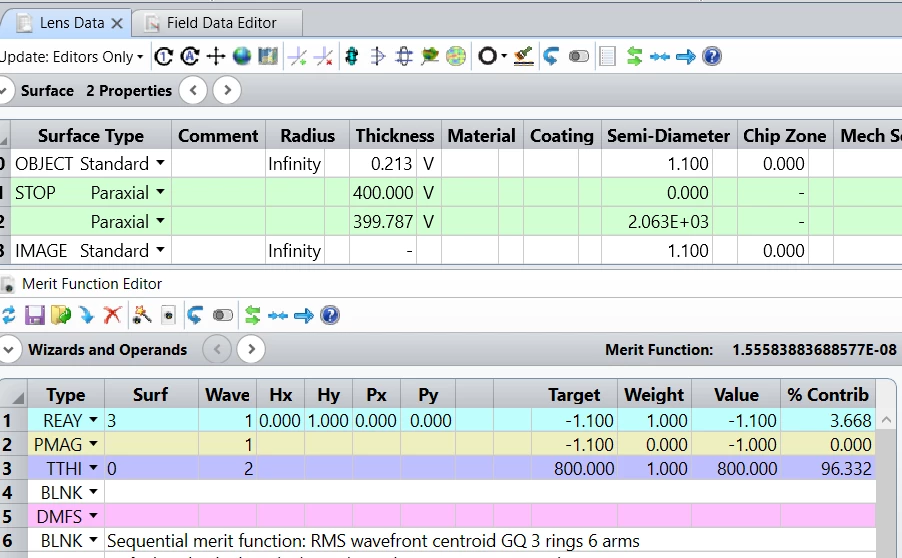# Paraxial magnification

• 1075 views

• Single Emitter
• 0 replies

Hello,

I ould be really thankful for clarification how to achieve a paraxial Magnification.

So I have system of two paraxial lenses with focuses 100 and 200. And I need to determince distance beween lenses and distances from lenses to the object and image. The magnification is M=-1.1x and total length of the system is 800mm.

So what I did. I definedthe obeject height 1mm in Fields and in merit function determined operand REAY with Py=1 and target=-1.1 to receive Magnification -1.1. But afer the optimization Paraxial Magnification less or higher than desired -1.1x.

So how is it possible to achieve the exact magnification M=-1.1x?Userlevel 5+3

Hi Anastasiia,

In your merit function, the REAY operand has Hx=0, Hy=0, Px=0, Py=0. This defines a ray starting at the (0,0) field position, which is on-axis, and traveling through the edge of the pupil. This is not what you want. You want to start the ray at the y=1 height so Hx=0, Hy=1, and send it through the center of the pupil, so Px=0, Py=0. (Note that Hy=1 because the normalized field is 1 for the ray height of 1mm.) Yout then target that ray to land at y = -1.1mm for a magnification of -1.1.

Note that you could also use the operand PMAG for paraxial magnification. (AMAG is angular magnification -- we want PMAG for a focal sysytem.)

It is worth noticing that is we weren't using paraxial lenses, the REAY would not necessarily result in a -1.1mm strike on the image plane because that is not a paraxial ray.

It's also worth noting that the optimizaton made the distance to the first lens nearly zero. This means the power contributed by that lens is negligible -- it could be eliminated from the design.

Kind regards,

David